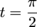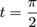Study Guide

# Word Problems

## Word Problems

Word problems involving integrals usually fall into one of two general categories: alien related and non-alien related. The non-alien related ones are totally the worst. Here's how to figure them out.

1) The problem may state in words what you're supposed to find, in which case you have to translate those words into symbols.

### Sample Problem

A snail travels with velocity

v(t) = 2 + 0.5sin t

feet per second, where t is given in seconds. To the nearest foot, how far does the snail travel from timeseconds to time t = 2π seconds?

The problem gives us a function for velocity and asks for distance travelled over a specified time interval. This means we need to use an integral.

To get the distance the snail travels, we integrate the velocity function over the specified time interval.Fromto t = 2π seconds, the snail travels approximately 9 feet.

2) Alternately, the problem could provide the symbols and ask you to translate back into words.

Problems where you need to translate from English into integrals can get a little more complicated.

Instead of the words translating into an integral you need to evaluate, the words might translate into an equation you need to solve. Such an equation will probably involve an integral with letters in the integrand or in the limits of integration.